Next: Normalization Using Join Dependencies Up: Normalization Using Multivalued Dependencies Previous: Theory of Multivalued Dependencies

## Fourth Normal Form (4NF)

1. We saw that BC-schema was in BCNF, but still was not an ideal design as it suffered from repetition of information. We had the multivalued dependency cnamestreet ccity, but no non-trivial functional dependencies.
2. We can use the given multivalued dependencies to improve the database design by decomposing it into fourth normal form.
3. A relation schema R is in 4NF with respect to a set D of functional and multivalued dependencies if for all multivalued dependencies in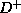of the form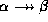, where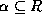and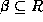, at least one of the following hold:
•is a trivial multivalued dependency.
•is a superkey for schema R.
4. A database design is in 4NF if each member of the set of relation schemas is in 4NF.
5. The definition of 4NF differs from the BCNF definition only in the use of multivalued dependencies.
• Every 4NF schema is also in BCNF.
• To see why, note that if a schema is not in BCNF, there is a non-trivial functional dependencyholding on R, whereis not a superkey.

• Sinceimplies, by the replication rule, R cannot be in 4NF.
6. We have an algorithm similar to the BCNF algorithm for decomposing a schema into 4NF:

``` result :=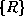;

done := false;

compute;

while (not done) do

if (there is a schema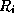in result

that is not in 4NF)

then begin

letbe a nontrivial multivalued

dependency that holds onsuch that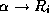is not in, and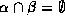;

result =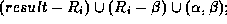end

else done = true;

```

7. If we apply this algorithm to BC-schema:
• cnameloan# is a nontrivial multivalued dependency and cname is not a superkey for the schema.

• We then replace BC-schema by two schemas:

``` Cust-loan-schema=(cname, loan#)

Customer-schema=(cname, street, ccity)

```

• These two schemas are in 4NF.
8. We can show that our algorithm generates only lossless-join decompositions.
• Let R be a relation schema and D a set of functional and multivalued dependencies on R.
• Let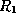and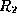form a decomposition of R.
• This decomposition is lossless-join if and only if at least one of the following multivalued dependencies is in:

```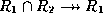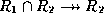```

• We saw similar criteria for functional dependencies.
• This says that for every lossless-join decomposition of R into two schemasand, one of the two above dependencies must hold.
• You can see, by inspecting the algorithm, that this must be the case for every decomposition.
9. Dependency preservation is not as simple to determine as with functional dependencies.
• Let R be a relation schema.
• Let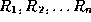be a decomposition of R.
• Let D be the set of functional and multivalued dependencies holding on R.
• The restriction of D tois the setconsisting of:
• All functional dependencies inthat include only attributes of.
• All multivalued dependencies of the form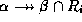where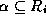andis in.
• A decomposition of schema R is dependency preserving with respect to a set D of functional and multivalued dependencies if for every set of relations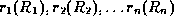such that for all i,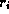satisfies, there exists a relation r(R) that satisfies D and for which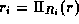for all i.
10. What does this formal statement say? It says that a decomposition is dependency preserving if for every set of relations on the decomposition schema satisfying only the restrictions on D there exists a relation r on the entire schema R that the decomposed schemas can be derived from, and that r also satisfies the functional and multivalued dependencies.
11. We'll do an example using our decomposition algorithm and check the result for dependency preservation.
• Let R=(A,B,C,G,H,I).
• Let D be

```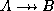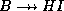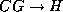```

• R is not in 4NF, as we haveand A is not a superkey.
• The algorithm causes us to decompose using this dependency into

```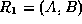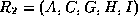```

•is now in 4NF, butis not.
• Applying the multivalued dependency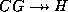(how did we get this?), our algorithm then decomposesinto

```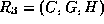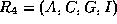```

•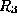is now in 4NF, but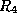is not.
• Why? As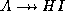is in(why?) then the restriction of this dependency togives us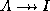.
• Applying this dependency in our algorithm finally decomposesinto

```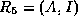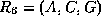```

• The algorithm terminates, and our decomposition is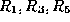and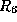.
12. Let's analyze the result.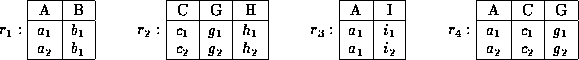Figure 7.9:   Projection of relation r onto a 4NF decomposition of R.

• This decomposition is not dependency preserving as it fails to preserve.
• Figure 7.9 (textbook 6.14) shows four relations that may result from projecting a relation onto the four schemas of our decomposition.
• The restriction of D to (A,B) isand some trivial dependencies.
• We can see that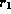satisfiesas there are no pairs with the same A value.
• Also,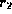satisfies all functional and multivalued dependencies since no two tuples have the same value on any attribute.
• We can say the same for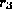and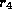.
• So our decomposed version satisfies all the dependencies in the restriction of D.
• However, there is no relation r on (A,B,C,G,H,I) that satisfies D and decomposes into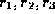and.
• Figure 7.10 (textbook 6.15) shows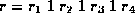.
• Relation r does not satisfy.
• Any relation s containing r and satisfyingmust include the tuple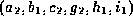.
• However,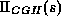includes a tuple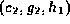that is not in.
• Thus our decomposition fails to detect a violation of.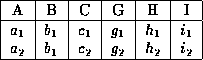Figure 7.10:   A relation r(R) that does not satisfy.

13. We have seen that if we are given a set of functional and multivalued dependencies, it is best to find a database design that meets the three criteria:
• 4NF.
• Dependency Preservation.
• Lossless-join.
14. If we only have functional dependencies, the first criteria is just BCNF.
15. We cannot always meet all three criteria. When this occurs, we compromise on 4NF, and accept BCNF, or even 3NF if necessary, to ensure dependency preservation.Next: Normalization Using Join Dependencies Up: Normalization Using Multivalued Dependencies Previous: Theory of Multivalued Dependencies

Osmar Zaiane
Thu Jun 18 12:56:34 PDT 1998